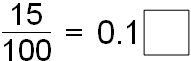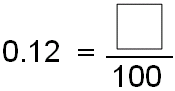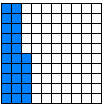Skill 25B
Decimals: Hundredths

1.

Write the fraction as a decimal.

Find the missing number.

Write the missing number in the box.2.

Write the decimal as a fraction.

Find the missing number.

Write the missing number in the box.3.

Find the missing numbers that the model show.

Write the missing numbers in the boxes.